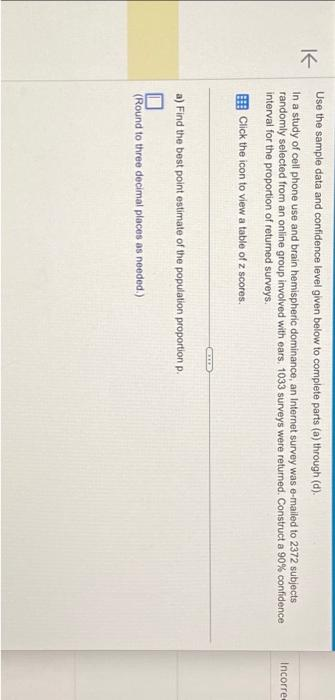Home / Expert Answers / Statistics and Probability / use-the-sample-data-and-confidence-level-given-below-to-complete-parts-a-through-d-in-a-study-pa787

# (Solved): Use the sample data and confidence level given below to complete parts (a) through (d). In a study ...Use the sample data and confidence level given below to complete parts (a) through (d). In a study of cell phone use and brain hemispheric dominance, an Internet survey was e-mailed to 2372 subjects randomly selected from an online group involved with ears, 1033 surveys were returned. Construct a confidence interval for the proportion of returned surveys. Click the icon to view a table of scores. a) Find the best point estimate of the population proportion p. (Round to three decimal places as needed.)

We have an Answer from Expert